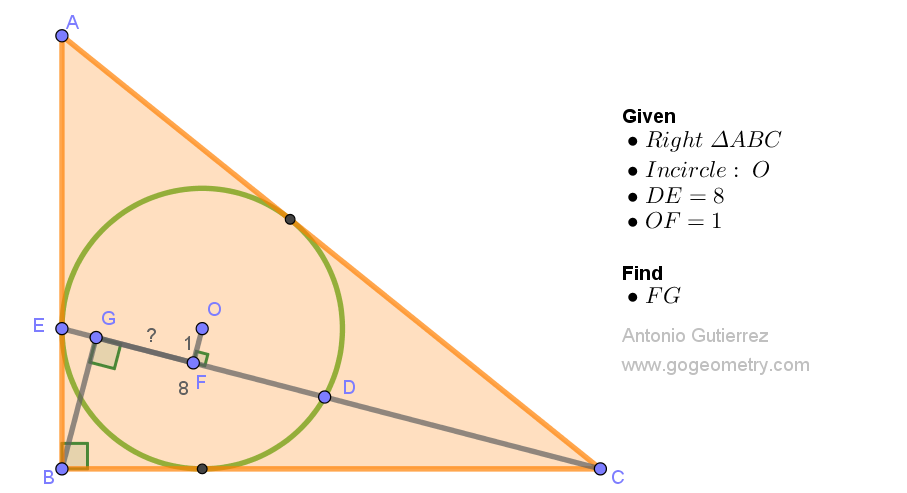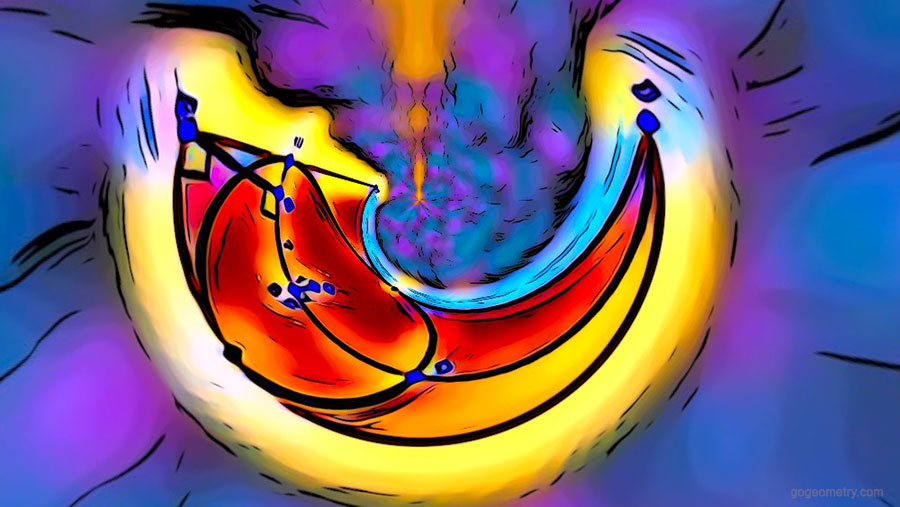# Geometry Problem 1556: Right Triangle ABC and Inscribed Circle. The problem involves circle, chords, tangent, perpendicular lines, and congruence

In a right triangle ABC, with the right angle at B, we have an inscribed circle centered at O. This circle touches AB at E and crosses CE at D. Next, we draw lines OF and BG perpendicular to CE. With DE measuring 8 units and OF measuring 1 unit, our aim is to find the length of FG.Triangle's silent tale,
Inscribed circle's gentle grace,
E and D converge,
Through lines OF and BG, we chase,
FG's length, the hidden trace.

## Utilizing Stereographic Projection in Geometric Art: Addressing Issue 1556In this illustration, stereographic projection and mathematical transformations are utilized to distort and reshape the geometry figure of problem 1554, producing distinctive spherical effects. This exemplifies an engaging application of geometry in digital image editing, empowering users to create visually captivating images from their figures.

Stereographic projection is a mathematical technique that maps points on a sphere to points on a plane (flat surface).

#### Problem 1551